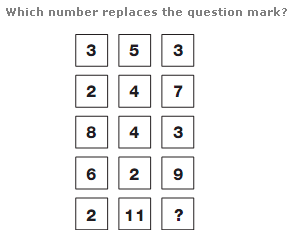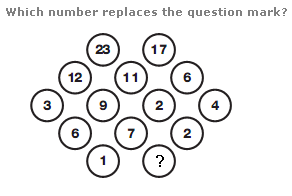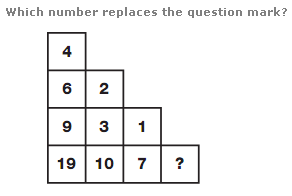# Puzzles - Number puzzles

### Exercise :: Number puzzlesAnswer : 6 Explanation : Calculating the sum of the 3 numbers in each row, from top to bottom, the rows follow the sequence of 11, 13, 15 etc.Answer : 5 Explanation : Start with the row of 4 numbers in the centre of the diagram, and add adjacent pairs of numbers together, putting the results in the circles directly above. Repeat this pattern for the next row up. Next, calculate the difference between adjacent numbers in the middle row, putting the results in the circles directly below. Repeat this pattern for the next row down.Answer : 6 Explanation : Start with the numbers along the top right diagonal of the diagram, and add pairs of adjacent numbers together, putting the result in the square to the lower left of the original pair of numbers. Continue in this way towards the lower left hand corner.